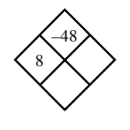### Home > MC1 > Chapter 4 > Lesson 4.1.4 > Problem4-47

4-47.

Copy and complete each of the Diamond Problems below. The pattern used in the Diamond Problems is shown at right.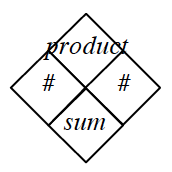1.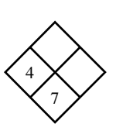Refer to problem MC1 2-108 for help if you are having trouble getting started.

To fill in the second $\#$ square, try to determine what plus $4$ is equal to $7$.

Next, fill in the product square by finding the product of $4$ and $3$.

$3$ and $12$ complete this diamond.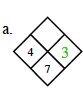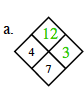1.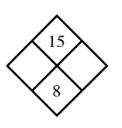What two numbers multiply to make $15$ and add together to make $8$?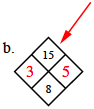1.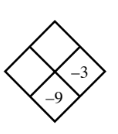For parts (c) and (d), follow the steps outlined in part (a). Depending on the problem, you can begin by either subtracting or dividing to find the second $\#$.

1.